Class 8 RD Sharma- Chapter 25 Data Handling III (Pictorial Representation Of Data As Pie Charts Or Circle Graphs) – Exercise 25.2

• Last Updated : 05 Nov, 2021

Question 1. The pie-chart given in the following represents the expenditure on different items in constructing a flat in Delhi. If the expenditure incurred on cement is Rs 112500, find the following: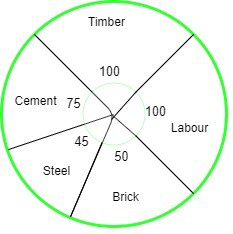(ii) Expenditure incurred on labour.

Solution:

(i) Expenditure incurred on cement =x total cost

⇒ 112500 =x total cost

Hence, the total cost of the flat = 540000

(ii) Expenditure incurred on labour =x total costx 540000

Hence, the expenditure incurred on labour = 150000

Question 2. The pie-chart given in the following shows the annual agricultural production of an Indian state. If the total production of all the commodities is 81000 tonnes, find the production (in tonnes) of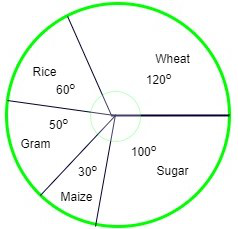(i) Wheat (ii) Sugar (iii) Rice (iv) Maize (v) Gram

Solution:

We are given total production = 81000

(i) Production (in tonnes) of wheat =x total productionx 81000

= 27000

(ii) Production (in tonnes) of sugar =x total productionx 81000

= 22500

(iii) Production (in tonnes) of rice =x total productionx 81000

= 13500

(iv) Production (in tonnes) of maize =x total productionx 81000

= 6750

(v) Production (in tonnes) of gram =x total productionx 81000

= 11250

Question 3. The following pie-chart shows the number of students admitted in different faculties of a college. If 1000 students are admitted in Science answer the following: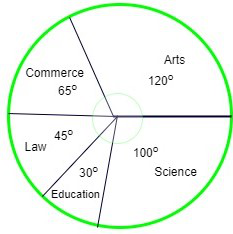(ii) What is the ratio of students in science and arts?

Solution:

(i) Students admitted in Science =x total students

⇒ 1000 =x total students

Hence, the total number of students = 3600

(ii) Students admitted in arts =x total students

⇒ students admitted in arts =x 3600

= 1200

Therefore, the ratio of students in science and arts == 5 : 6

Question 4. In the following figure, the pie-chart shows the marks obtained by a student in an examination. If the student secures 440 marks in all, calculate his marks in each of the given subjects.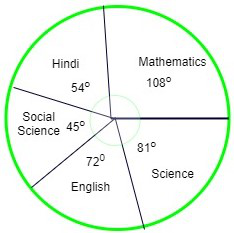Solution:

We are given total marks scored by student = 440

Marks of student in Mathematics =x total marksx 440 = 132

Marks of student in Hindi =x total marksx 440 = 66

Marks of student in Social Science =x total marksx 440 = 55

Marks of student in English =x total marksx 440 = 88

Marks of student in Science =x total marksx 440 = 99

Question 5. In the following figure, the pie-chart shows the marks obtained by a student in various subjects. If the student scored 135 marks in mathematics, find the total marks in all the subjects. Also, find his score in individual subjects.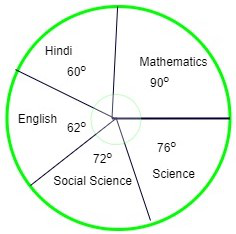Solution:

Marks scored in Mathematics =x total marks

⇒ 135 =x total marks

⇒ total marks scored by student = 540

Marks of student in Hindi =x total marksx 540 = 90

Marks of student in Social Science =x total marksx 540 = 108

Marks of student in English =x total marksx 540 = 93

Marks of student in Science =x total marksx 540 = 114

Question 6. The following pie-chart shows the monthly expenditure of Shikha on various items. If she spends Rs 16000 per month, answer the following questions: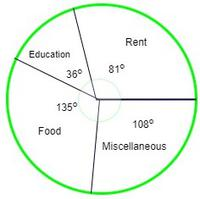(iii) What is the ratio of expenses on food and rent?

Solution:

Given, total money spent by Shikha = Rs. 16000

(i) Expenditure on rent =x total moneyx 16000

Hence, the total spent on rent = 3600

(ii) Expenditure on education =x total costx 16000

Hence, the total spent on education = 1600

(iii) Expenditure on food =x total costx 16000

Hence, the total spent on food = 6000

Therefore, the ratio of expenses on food and rent == 5 : 3

Question 7. The pie chart (as shown in the figure) represents the amount spent on different sports by a sports club in a year. If the total money spent by the club on sports is Rs 1,08,000, find the amount spent on each sport.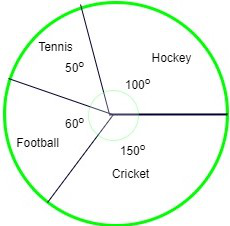Solution:

Given, total money spent by the club on sports = Rs 1,08,000

Amount spent on Tennis =x total moneyx 108000

Hence, the total amount spent on Tennis = 15000

Amount spent on Hockey =x total moneyx 108000

Hence, the total amount spent on Hockey = 30000

Amount spent on Football =x total moneyx 108000

Hence, the total amount spent on Football = 18000

Amount spent on Cricket =x total moneyx 108000

Hence, the total amount spent on Cricket = 45000

My Personal Notes arrow_drop_up